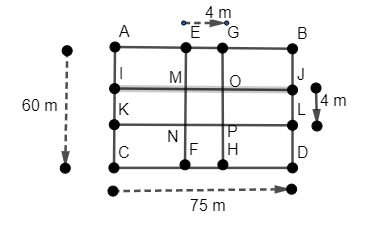QuestionAnswers

# A rectangular lawn 75 m by 60 m, has two roads 4 m wide each running through the middle of the lawn one parallel to the length and other parallel to the breath as shown in figure. Find the cost of graveling the roads at Rs. 4.50 per sq m.A. Rs. 2358B. Rs. 2385C. Rs. 2300D. Rs. 2500Hint: For finding the cost of gravelling, first we have to find the total area of road (area of the rectangle IJLK + area of rectangle EFHG – area of square MNPO). And by multiplying the area of road to the cost of graveling per square meter, we will get the total cost.
The area of rectangle is given by the formula = length $\times$ breadth
The area of square is given by the formula = side $\times$ side

Complete step by step solution:
The figure for the given problem is as follows:Now in the given question, it is given that
The length of the rectangular lawn is = 75 m
The breadth of the rectangular lawn is = 60 m
We have to find the cost of graveling the road which is inside the rectangle road for which the cost per sq m is 4.50 rupees.
So for that we have to find the area of the road and multiply it with 4.50.
The area of the road is given by
= area of the rectangle IJLK + area of rectangle EFHG – area of square MNPO …….(1)
The area of rectangle is given by the formula = length $\times$ breadth
The length of JL is given = 4 m
And the length of KL as it is parallel to line AB or CD = 75 m
So the area of rectangle IJLK = length of JL $\times$ length of KL
$\Rightarrow 4\times 75=300{{m}^{2}}.......(2)$
The length of EG = 4 m
The length of EF as it is parallel to line AC or BD = 60 m
So the area of EFHG = length of EG $\times$ length of EF
$\Rightarrow 4\times 60=240{{m}^{2}}.........(3)$
The area of square is given by the formula = side $\times$ side
The side of square MNPO = 4 m
So the area of square MNPO is equal to
$\Rightarrow 4\times 4=16{{m}^{2}}......(4)$
Now using equation (1), (2), (3) and (4), we get
= $300+240-16=524{{m}^{2}}$
Now we are given that the cost of gravelling for 1 sq m is 4.50 Rs.
Cost of the gravelling for the road is = total area of road $\times$ cost of gravelling for one sq m
That is
= $524\times 4.5=2358$
So the cost for graveling of the road is 2358 Rs.
Hence the correct option is (A).

Note: Students may make the mistake that they do not subtract the area of square MNPO from the area of rectangle IJLK + area of rectangle EFHG. Because square MNPO is common in both rectangles IJLK and EFHG.
Table of 75 - Multiplication Table of 75Table of 60 - Multiplication Table of 60Area of a Sector of a Circle FormulaArea of a Rhombus FormulaSurface Area of a Prism FormulaCBSE Class 9 Maths FormulasTo Determine the Mass of Two Different Objects Using a Beam BalanceTable of 4 - Multiplication Table of 4Table of 9 - Multiplication Table of 9Integration By Parts FormulaImportant Questions for CBSE Class 9 Maths Chapter 4 - Linear Equations in Two VariablesNCERT Books for Class 9 Maths Chapter 4Important Questions for CBSE Class 6 English A Pact with The Sun Chapter 1 - A Tale of Two BirdsImportant Questions for CBSE Class 9 Science Chapter 4 - Structure of The AtomNCERT Books for Class 9 Science Chapter 4Important Questions for CBSE Class 11 Physics Chapter 4 - Motion in a PlaneImportant Questions for CBSE Class 7 English Honeycomb Chapter 9 - A Bicycle In Good RepairImportant Questions for CBSE Class 9 Maths Chapter 9 - Areas of Parallelograms and TrianglesImportant Questions for CBSE Class 9 MathsNCERT Books for Class 9 Maths Chapter 9CBSE Class 10 Hindi A Question Paper 2020Hindi A Class 10 CBSE Question Paper 2009Hindi A Class 10 CBSE Question Paper 2015Hindi A Class 10 CBSE Question Paper 2016Hindi A Class 10 CBSE Question Paper 2012Hindi A Class 10 CBSE Question Paper 2010Hindi A Class 10 CBSE Question Paper 2007Hindi A Class 10 CBSE Question Paper 2013Hindi A Class 10 CBSE Question Paper 2008Hindi A Class 10 CBSE Question Paper 2014NCERT Solutions for Class 9 Maths Chapter 4 Linear Equations in Two VariablesRD Sharma Class 9 Solutions Chapter 4 - Algebraic Identities (Ex 4.4) Exercise 4.4NCERT Solutions for Class 9 Maths Chapter 4 Linear Equations in Two Variables (Ex 4.4) Exercise 4.4NCERT Solutions for Class 9 Maths Chapter 9 Areas of Parallelograms and Triangles (Ex 9.4) Exercise 9.4RD Sharma Class 9 Solutions Chapter 4 - Algebraic Identities (Ex 4.1) Exercise 4.1RD Sharma Class 8 Solutions Chapter 9 - Linear Equation In One Variable (Ex 9.4) Exercise 9.4RD Sharma Class 6 Solutions Chapter 9 - Ratio, Proportion and Unitary Method (Ex 9.4) Exercise 9.4RS Aggarwal Solutions Class 10 Chapter 9 - Mean, Median & Mode (Ex 9D) Exercise 9.4RD Sharma Class 10 Solutions Chapter 9 - Exercise 9.4RD Sharma Class 9 Solutions Chapter 4 - Algebraic Identities (Ex 4.2) Exercise 4.2